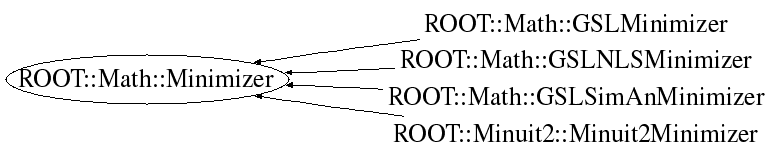# class ROOT::Math::Minimizer

```
Abstract Minimizer class, defining  the interface for the various minimizer
(like Minuit2, Minuit, GSL, etc..)
Plug-in's exist in ROOT to be able to instantiate the derived classes like
ROOT::Math::GSLMinimizer or ROOT::Math::Minuit2Minimizer via the
plug-in manager.

Provides interface for setting the function to be minimized.
The function must  implemente the multi-dimensional generic interface
ROOT::Math::IBaseFunctionMultiDim.
If the function provides gradient calculation
(implements the ROOT::Math::IGradientFunctionMultiDim interface) this will be
used by the Minimizer.

It Defines also interface for setting the initial values for the function variables (which are the parameters in
of the model function in case of solving for fitting) and especifying their limits.

It defines the interface to set and retrieve basic minimization parameters
(for specific Minimizer parameters one must use the derived classes).

Then it defines the interface to retrieve the result of minimization ( minimum X values, function value,
gradient, error on the mimnimum, etc...)

@ingroup MultiMin

```

## Function Members (Methods)

This is an abstract class, constructors will not be documented.
Look at the header to check for available constructors.

public:
 virtual ~Minimizer() virtual void Clear() virtual double CovMatrix(unsigned int i, unsigned int j) const virtual double Edm() const virtual const double* Errors() const double ErrorUp() const virtual bool GetMinosError(unsigned int, double& errLow, double& errUp) unsigned int MaxFunctionCalls() unsigned int MaxIterations() virtual const double* MinGradient() const virtual bool Minimize() virtual double MinValue() const virtual unsigned int NCalls() const virtual unsigned int NDim() const virtual unsigned int NFree() const int PrintLevel() const virtual bool ProvidesError() const void SetErrorUp(double up) virtual bool SetFixedVariable(unsigned int ivar, const string& name, double val) virtual void SetFunction(const ROOT::Math::Minimizer::IObjFunction& func) virtual void SetFunction(const ROOT::Math::Minimizer::IGradObjFunction& func) virtual bool SetLimitedVariable(unsigned int ivar, const string& name, double val, double step, double, double) virtual bool SetLowerLimitedVariable(unsigned int ivar, const string& name, double val, double step, double lower) void SetMaxFunctionCalls(unsigned int maxfcn) void SetMaxIterations(unsigned int maxiter) void SetPrintLevel(int level) void SetStrategy(int strategyLevel) void SetTolerance(double tol) virtual bool SetUpperLimitedVariable(unsigned int ivar, const string& name, double val, double step, double upper) virtual bool SetVariable(unsigned int ivar, const string& name, double val, double step) int Strategy() const double Tolerance() const virtual const double* X() const
private:
 ROOT::Math::Minimizer& operator=(const ROOT::Math::Minimizer& rhs)

## Data Members

protected:
 int fDebug print level unsigned int fMaxCalls max number of funciton calls unsigned int fMaxIter max number or iterations used to find the minimum int fStrategy minimizer strategy double fTol tolerance (absolute) double fUp error scale

## Class Charts## Function documentation

virtual ~Minimizer()
```Destructor (no operations)

```
`{}`
void Clear()
``` reset for consecutive minimizations - implement if needed
```
`{}`
void SetFunction(const IObjFunction & func)
``` set the function to minimize
```
``` set a function to minimize using gradient
```
bool SetVariable(unsigned int ivar, const string& name, double val, double step)
``` set free variable
```
bool SetLowerLimitedVariable(unsigned int ivar, const string& name, double val, double step, double lower)
return SetLimitedVariable(unsigned int ivar, const string& name, double val, double step, double , double )
bool SetUpperLimitedVariable(unsigned int ivar, const string& name, double val, double step, double upper)
``` set upper limit variable (override if minimizer supports them )
```
bool SetFixedVariable(unsigned int ivar, const string& name, double val)
``` set fixed variable (override if minimizer supports them )
```
bool Minimize()
``` method to perform the minimization
```
double MinValue()
``` return minimum function value
```
double Edm()
``` return expected distance reached from the minimum
```
const double * X()
``` return  pointer to X values at the minimum
```
``` return pointer to gradient values at the minimum
```
unsigned int NCalls()
``` number of function calls to reach the minimum
```
unsigned int NDim()
``` this is <= Function().NDim() which is the total
number of variables (free+ constrained ones)
```
unsigned int NFree()
``` number of free variables (real dimension of the problem)
this is <= Function().NDim() which is the total
```
bool ProvidesError()
``` minimizer provides error and error matrix
```
const double * Errors()
``` return errors at the minimum
```
double CovMatrix(unsigned int i, unsigned int j) const
``` return covariance matrices elements
if the variable is fixed the matrix is zero
The ordering of the variables is the same as in errors

```
bool GetMinosError(unsigned int , double& errLow, double& errUp)
``` minos error for variable i, return false if Minos failed or not supported
```
int PrintLevel()
``` return reference to the objective function
virtual const ROOT::Math::IGenFunction & Function() const = 0;
minimizer configuration parameters
set print level
```
`{ return fDebug; }`
unsigned int MaxFunctionCalls()
```  max number of function calls
```
`{ return fMaxCalls; }`
unsigned int MaxIterations()
``` max iterations
```
`{ return fMaxIter; }`
double Tolerance()
``` absolute tolerance
```
`{ return fTol; }`
int Strategy()
``` strategy
```
`{ return fStrategy; }`
double ErrorUp()
``` return the statistical scale used for calculate the error
is typically 1 for Chi2 minimizetion and 0.5 for likelihood's
```
`{ return fUp; }`
void SetPrintLevel(int level)
``` set print level
```
`{ fDebug = level; }`
void SetMaxFunctionCalls(unsigned int maxfcn)
```set maximum of function calls
```
`{ if (maxfcn > 0) fMaxCalls = maxfcn; }`
void SetMaxIterations(unsigned int maxiter)
``` set maximum iterations (one iteration can have many function calls)
```
`{ if (maxiter > 0) fMaxIter = maxiter; }`
void SetTolerance(double tol)
``` set the tolerance
```
`{ fTol = tol; }`
void SetStrategy(int strategyLevel)
```set the strategy
```
`{ fStrategy = strategyLevel; }`
void SetErrorUp(double up)
``` set scale for calculating the errors
```
`{ fUp = up; }`

Author: L. Moneta Fri Sep 22 15:06:47 2006
Last update: root/mathcore:\$Id: Minimizer.h 21503 2007-12-19 17:34:54Z moneta \$
Copyright (c) 2006 LCG ROOT Math Team, CERN/PH-SFT *

This page has been automatically generated. If you have any comments or suggestions about the page layout send a mail to ROOT support, or contact the developers with any questions or problems regarding ROOT.